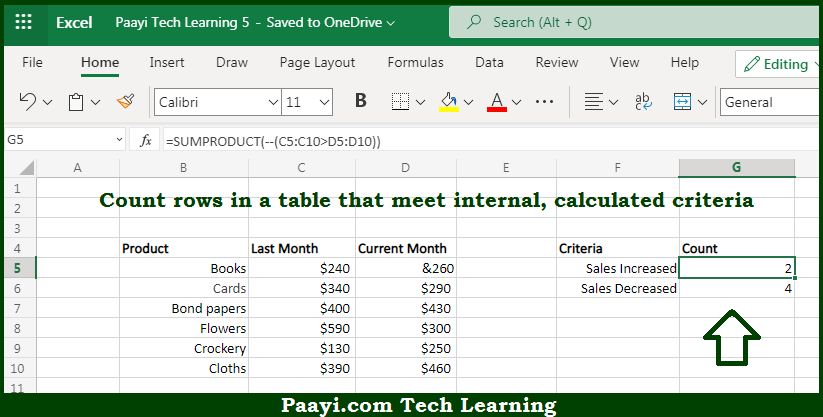# Learn How to Count if Row Meets Internal Criteria in Microsoft Excel

Written by | 0 Comments | 567 Views

In this article, you will learn how to COUNT various things in Microsoft Excel using a single or combination of functions and its purpose in Microsoft Excel. You will also get to know how to Count if Row Meets Internal Criteria.

Count if Row Meets Internal Criteria in Microsoft Excel

The main purpose of this formula is to count rows in a given table that meets the internal, calculated criteria. Here we will learn how to count rows that meet internal criteria in Microsoft Excel. That implies, with the help of a formula based on the SUMPRODUCT function you can able to count rows in a table that meet internal, calculated criteria, without using a helper column. So, with the help of this formula, you can able to count rows that meet internal criteria.

General Formula to Count if Row Meets Internal Criteria

=SUMPRODUCT(--(logical_expression))

The Explanation for the Count if Row Meets Internal CriteriaSo we know that with the help of the given formula above you can able to count rows in a given table that meets the internal, calculated criteria. Here we will learn how to count rows that meet internal criteria in Microsoft Excel. As we know that, the SUMPRODUCT's main purpose is to work smoothly with arrays. It multiplies corresponding elements in two or more arrays and sums the resulting products. That is why you can use the SUMPRODUCT function to process arrays that result from criteria being applied to a range of cells. It should be noted that the result of such operations will be arrays, which the SUMPRODUCT function can handle efficiently. So, with the help of this formula, you can able to count rows in a given table that meets the internal, calculated criteria.